# Ernst equation

In an oriented space-time with a time-like Killing vector field, the twist-formis defined by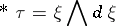where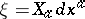. It is always closed in vacuum solutions to the Einstein gravitational equations; that is, when the Ricci tensor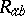vanishes.

In such space-times, one can write (locally)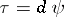, whereis constant along. The Ernst potential is the complex quantity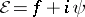, where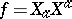and. It is used in a number of different ways in finding explicit solutions to Einstein's equations (cf. also Einstein equations; [a8] provides a wide-ranging introduction to the most of the original work on this subject).

One use is in the generation of new solutions with one Killing vector from a known one. The idea here is to use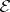and the metricon the quotient space by the Killing vector action as dependent variables (both are functions of three variables). The vacuum equations for the space-time metric can then be derived from the actionwhereandare the scalar curvature and the volume element of the-metric. There is a straightforward extension to the Einstein–Maxwell equations.

The symmetries of the action and its electro-magnetic generalization allow transformations of the solution that preserve, but change the potential. They include solution-generation transformations discussed in [a6], [a7].

A second use is in finding stationary axi-symmetric gravitational fields (or by a straightforward modification to the formalism, solutions with other symmetries representing, for example, cylindrically symmetric gravitational waves and the interaction of colliding plane waves). Here one assumes the existence a second Killing vectorsuch thatandtogether generate a-dimensional Lie algebra of infinitesimal isometries. In this case, there are two twist-forms. Their inner products with the Killing vectors are constant when, and vanish if some combination of the Killing vectors has a fixed point.

When they do vanish, the space-time metric can be written in the Weyl canonical form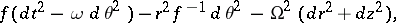where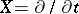and. In this case, the Ernst potential associated withis a function ofandalone, and the vacuum equations reduce to the Ernst equation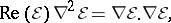where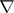is the gradient in the three-dimensional Euclidean space on which,,are cylindrical polar coordinates [a4]. Onceis known,is found by quadrature. Again, there is a straightforward extension to the Einstein–Maxwell case [a5].

Although still non-linear, this reduction to a single scalar equation in Euclidean space for the complex potentialis a great simplification of the original vacuum equations. It has been widely exploited in the search for exact solutions. In particular, the solution-generation techniques provide a rich source of new solutions since one can combine the transformations of a metric with one Killing vector with linear transformations in the Lie algebra spanned byand.

Although it is non-linear, the Ernst equation is integrable, and its transformation properties can be seen as part of the wider theory of integrable systems (cf. also Integrable system); some of the connections are explained in [a3]. One can understand them from another point of view through the observation [a2] that the Ernst equation is identical to a form of the self-dual Yang–Mills equation (cf. also Yang–Mills field) for static axi-symmetric gauge fields. If one writes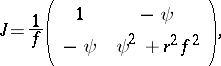then the Ernst equation is equivalent to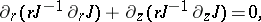which is a symmetry reduction of the Yang equation. Solutions can therefore be found by solving a Riemann–Hilbert problem [a10], and, more generally, by the twistor methods reviewed in [a9].

The space-time metric gives rise to a solution of this same equation in another way by writingThe mapping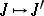is a discrete symmetry of the reduction of Yang's equation, and many of the solution transformations can be obtained by combining it with,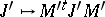for constant matricesand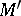. In [a1], these are seen to generate the action of a loop group (in fact a central extension when the action on the conformal factoris included).